# How to write a infinity symbol(∞) in LaTeX?

Usually, you are all familiar with the infinity symbol. And use of this infinity symbol is much more in mathematics. Mathematically, infinity is denoted by the symbol.

 Properties Value Symbol Infinity Argument No Command \infty Example \infty → ∞

However, to represent this infinity symbol in latex, you need to use the \infty command.

\documentclass{article}
\begin{document}
$$\left ( -\infty ,+\infty \right )$$
\end{document}

Output :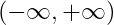## List of indeterminate forms in LaTeX

The Indeterminate forms of Infinity are discussed with the help of the table below

 1^{\infty}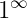\infty^{0}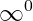\frac{\infty}{\infty}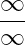0\cdot\infty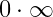\infty-\infty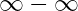Don’t be confused to use single dollar and double dollar on both sides of the equation.

If you want to use inline equations. Then you need to add single dollar sign on both sides of latex equation.

\documentclass{article}
\begin{document}
Range of R $\left(-infty,0 \right]$
\end{document}

Output :

Range of R (-∞,0]

And if you write the equation separately instead of using the text, you can use the double dollar sign on both sides of the equation.

\documentclass{article}
\begin{document}
Range of R $\left(-infty,0 \right]$
$$\lim_{x \to \infty}f(x)$$
\end{documeet}

Output :

Range of R (-∞,0]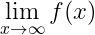## Use of Infinity Symbol in Mathematics

1. This infinity symbol is used when determining the domain and range of an expression.

\documentclass{article}
\begin{document}
$R \in (-\infty,a]\cup[b,+\infty)$
\end{document}

Output :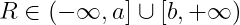And if you look at the code above, you can see that the \in command has been used for the  symbol.

2. Also, the infinity symbol is used to represent the limit. And in this case, plus or minus is used before the limit.

\documentclass{article}
\begin{document}
$$\lim_{x\to\infty}=f(x)$$
$$\lim_{x\to+\infty}=\frac{1}{x}$$
$$\lim_{x\to-\infty}=\frac{x+1}{x-1}$$
\end{document}

Output :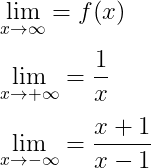3. The Infinity symbol is used when writing the Infinity series with a summation symbol. Notice this program below

\documentclass{article}
\begin{document}
$$\sum_{n=1}^{\infty} \frac{1}{n}$$
$$\sum_{n=1}^{\infty} \frac{1}{n+1}$$
\end{document}

Output :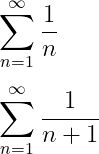4. And if you have read Quantum Physics, you will see that this infinity symbol is also used to define different equations.

\documentclass{article}
\begin{document}
$\int_{-\infty}^{\infty}e^{-ax^{2}}\:dx=\sqrt{\frac{\pi}{a}}$
\end{document}

Output :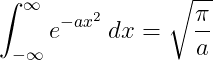And you may see in many documents that the negative infinity symbol is used. In this case, you need to use the minus symbol before the latex \infty command.

### 2 thoughts on “How to write a infinity symbol(∞) in LaTeX?”

1.\infty works for me, but not \infity

1.Thanks Jon, for correction. It was a spelling mistake by me. And now I am correcting this mistake.